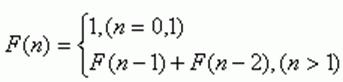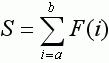favorite We need a little bit of your help to keep things running, click on this banner to learn more
Competitions

# Fibonacci Problem Again

As we know, the Fibonacci numbers are defined as follows::Given two numbers a and b, calculate.

#### Input

Consists of several test cases. Each test case is a separate line with two non-negative integers a and b (0ab`109`).

#### Output

For each test case output S mod `109`, since S may be quite large.

Time limit 1 second
Memory limit 128 MiB
Input example #1
```1 1
3 5
10 1000
```
Output example #1
```1
16
496035733
```